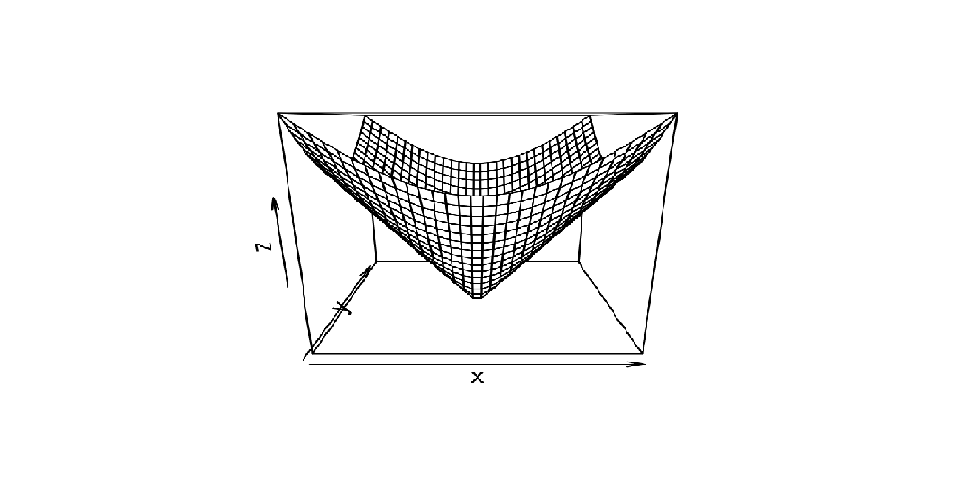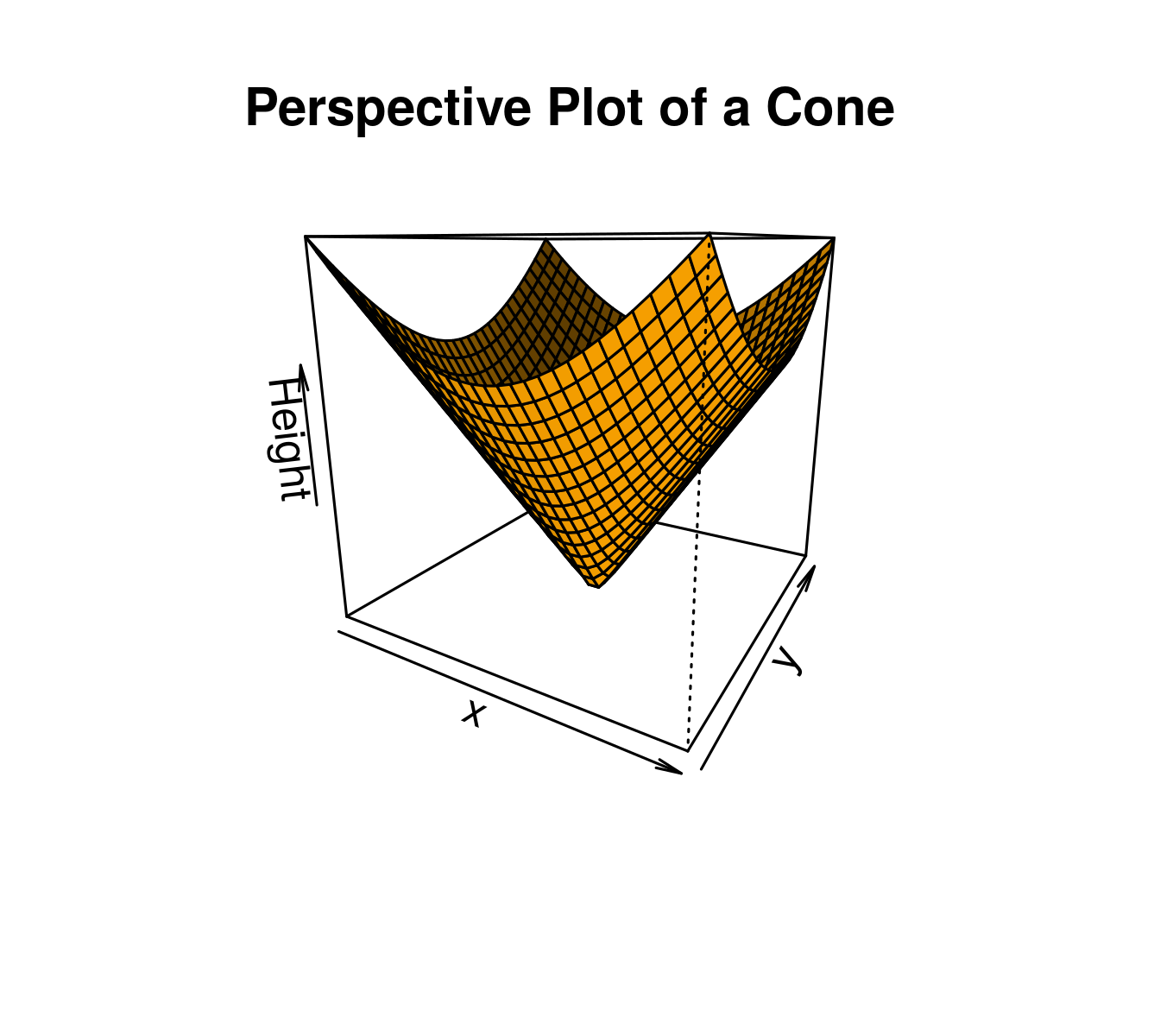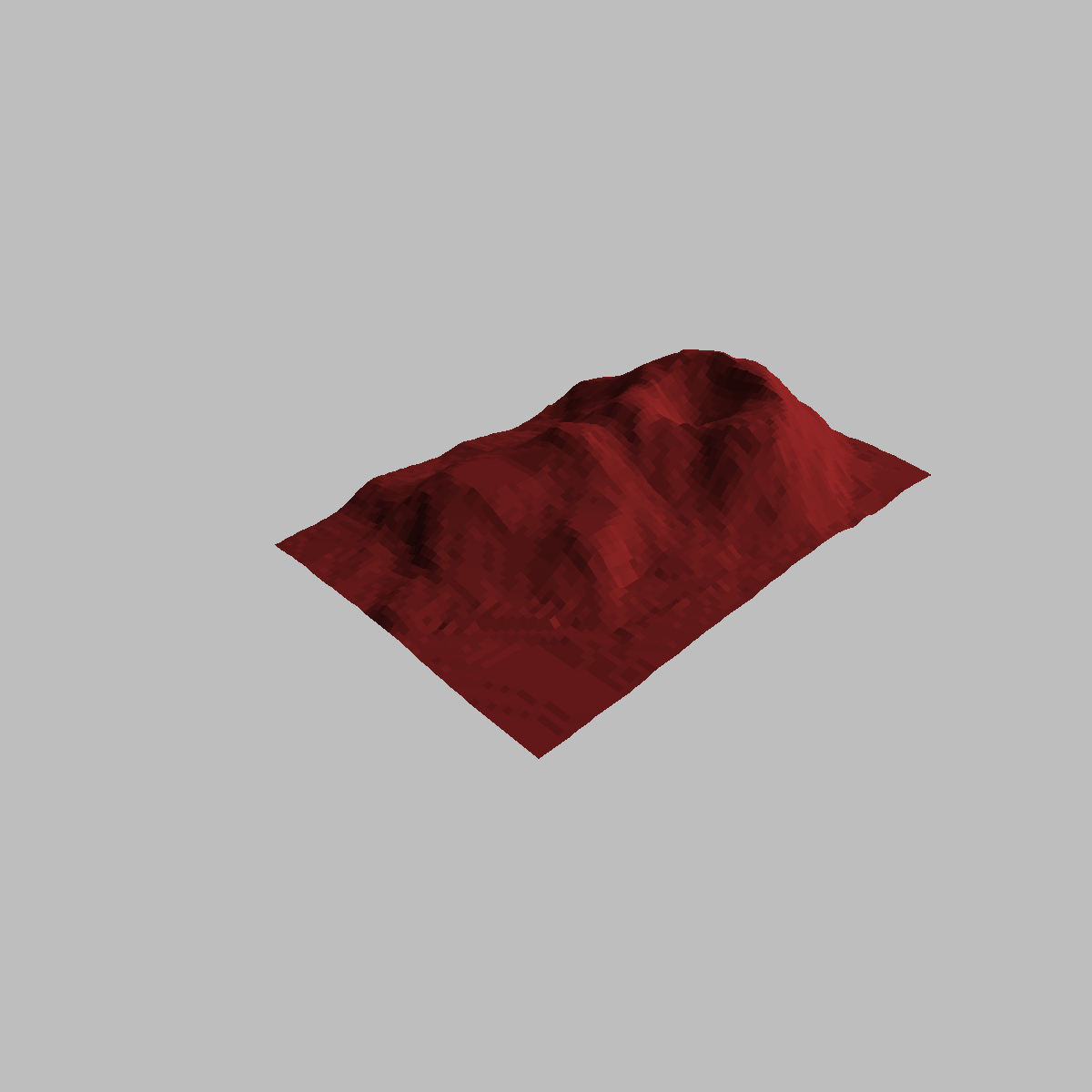Related Articles

# Creating 3D Plots in R Programming – persp() Function

• Last Updated : 08 Jun, 2020

3D plot in R Language is used to add title, change viewing direction, and add color and shade to the plot. The `persp()` function which is used to create 3D surfaces in perspective view. This function will draw perspective plots of a surface over the x–y plane. `persp()` is defines as a generic function. Moreover, it can be used to superimpose additional graphical elements on the 3D plot, by `lines()` or `points()`, using the function `trans3d()`.

Syntax: persp(x, y, z)

Parameter: This function accepts different parameters i.e. x, y and z where x and y are vectors defining the location along x- and y-axis. z-axis will be the height of the surface in the matrix z.

Return Value: persp() returns the viewing transformation matrix for projecting 3D coordinates (x, y, z) into the 2D plane using homogeneous 4D coordinates (x, y, z, t).

Example 1: Simple Right Circular Cone

 `# To illustrate simple right circular cone``cone <``-` `function(x, y){``sqrt(x ^ ``2` `+` `y ^ ``2``)``}`` ` `# prepare variables.``x <``-` `y <``-` `seq(``-``1``, ``1``, length ``=` `30``)``z <``-` `outer(x, y, cone)`` ` `# plot the 3D surface``persp(x, y, z)`

Output:• Here in above code, function `seq()` to generate vector of equally spaced numbers.
• the `outer()` function to apply the function cone at every combination of x and y.

Example 2: Adding Titles and Labeling Axes to Plot

 `# Adding Titles and Labeling Axes to Plot``cone <``-` `function(x, y){``sqrt(x ^ ``2` `+` `y ^ ``2``)``}`` ` `# prepare variables.``x <``-` `y <``-` `seq(``-``1``, ``1``, length ``=` `30``)``z <``-` `outer(x, y, cone)`` ` `# plot the 3D surface``# Adding Titles and Labeling Axes to Plot``persp(x, y, z,``main``=``"Perspective Plot of a Cone"``,``zlab ``=` `"Height"``,``theta ``=` `30``, phi ``=` `15``,``col ``=` `"orange"``, shade ``=` `0.4``)`

Output:• Here in above code, xlab, ylab and zlab can be used to label the three axes.
• theta and phi are viewing direction.

Example 3: Visualizing a simple DEM(Digital elevation model)

 `# Visualizing a simple DEM model`` ` `z <``-` `2` `*` `volcano        ``# Exaggerate the relief``x <``-` `10` `*` `(``1``:nrow(z))   ``# 10 meter spacing (S to N)``y <``-` `10` `*` `(``1``:ncol(z))   ``# 10 meter spacing (E to W)`` ` ` ` `# Don't draw the grid lines :  border = NA``par(bg ``=` `"gray"``)``persp(x, y, z, theta ``=` `135``, phi ``=` `30``, col ``=` `"brown"``, scale ``=` `FALSE,``      ``ltheta ``=` `-``120``, shade ``=` `0.75``, border ``=` `NA, box ``=` `FALSE)`

Output:My Personal Notes arrow_drop_up